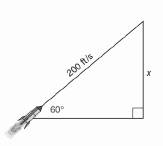Chapter 11.CR, Problem 22CR### Elementary Geometry for College St...

6th Edition
Daniel C. Alexander + 1 other
ISBN: 9781285195698

#### Solutions

Chapter
Section### Elementary Geometry for College St...

6th Edition
Daniel C. Alexander + 1 other
ISBN: 9781285195698
Textbook Problem
1 views

# In exercises 21 to 30. Use the drawings. Where provided, to solve each problem. Angle measures should be found to the nearest degree; lengths should be found to the nearest tenth of a unit.A rocket is shot into the air at angle of 60 ° If it is traveling at 200 ft per second, how high in the air is it after 5 seconds? (Ignoring gravity, assume that the path of the rocket is a straight line.)To determine

To find:

The height of the rocket in air after 5 seconds of its shot at an angle of 60° and it is travelling at 200 ft per second.

Explanation

Procedure used:

In any right triangle ABC with mA=θ we have the following ratios.

sinθ=OppositeHypotenuse

Calculation:

Given:

Speed of the rocket =200 ft per second.

Angle of projection (elevation) =60°

The height of the rocket in air after 5 seconds =x.

Let us structure the given figure as follows as a right triangle.

First let us find the distance travelled by the rocket in 5 second.

Distance travelled = Speed × Time

Distance travelled by the rocket

=200×5

=1000 ft

### Still sussing out bartleby?

Check out a sample textbook solution.

See a sample solution

#### The Solution to Your Study Problems

Bartleby provides explanations to thousands of textbook problems written by our experts, many with advanced degrees!

Get Started

#### In Exercises 516, evaluate the given quantity. log1/212

Finite Mathematics and Applied Calculus (MindTap Course List)

#### Factor: y3y2

Elementary Technical Mathematics

#### In Exercises 73-80, find the indicated limits, if they exist. 78. limx4x43x2+12x4+x3+x2+x+1

Applied Calculus for the Managerial, Life, and Social Sciences: A Brief Approach

#### Identify and describe the steps in the research process.

Research Methods for the Behavioral Sciences (MindTap Course List)